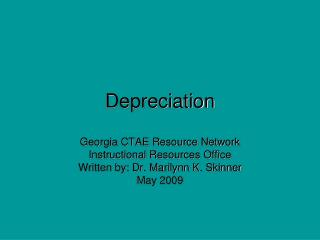DownloadDownload PresentationDepreciation

# Depreciation

Download Presentation## Depreciation

- - - - - - - - - - - - - - - - - - - - - - - - - - - E N D - - - - - - - - - - - - - - - - - - - - - - - - - - -
##### Presentation Transcript

1. Depreciation Georgia CTAE Resource Network Instructional Resources Office Written by: Dr. Marilynn K. Skinner May 2009

2. LEARNING GOALS • Define, classify, account for cost of fixed assets. • Compute depreciation using straight-line, units-of-production, declining balance. • Account for disposal of fixed assets. Continued

3. LG 1 Why is a “major purchase” different? Major purchase is expensive & long-lived.

4. LG 1 CLASSIFYING COSTS Expenditures must be classified as • Expenses • Investments • Fixed assets

5. LG 1 EXHIBIT 2 When is a purchased item a fixed asset? Yes No Expense No Yes Fixed Assets Investments

6. LEARNING GOALS 2 Compute depreciation using straight-line, units-of-production, declining balance.

7. LG 2 EXHIBIT 4

8. LG 2 What are some methods of depreciation? We examine 3 depreciation methods.

9. LG 2 DEPRECIATION METHODS 3 methods to calculate depreciation expense • Straight-line depreciation • Same amount of depreciation each year • Units of production depreciation • Depreciation varies with asset use • Declining balance • Accelerated method provides more depreciation in earlier years

10. LG 2 STRAIGHT-LINE DEPRECIATION Depreciation expense = (Cost – Residual value) / Useful life

11. LG 2 EXERCISE 9-15a Press “Enter” or click left mouse button for answer. A backhoe acquired on 1/5 for \$84,000 has an estimated useful life of 12 years and no residual value. Determine the depreciation for each of the first 2 years by straight-line depreciation. Click button to skip this exercise Depreciation expense = (Cost – Residual value)/ Useful life (\$84,000 - \$0)/12 = \$7,000 year 1 & 2

12. LG 2 UNITS-OF-PRODUCTION DEPRECIATION Depreciation expense = {(Cost – Residual value)/ Productive activity} * Current activity

13. LG 2 EXERCISE 9-13 A diesel-powered generator with cost of \$345,000 and residual value of \$18,000, has an expected operating life of 75,000 hours. In July, the generator was used 1,250 hours. Calculate depreciation by units-of-production depreciation. Press “Enter” or click left mouse button for answer. Click button to skip this exercise Depreciation expense = {(Cost – Residual value)/ Productive activity} * Current activity {(\$345,000 - \$18,000)/75,000} * 1,250 = \$5,450

14. LG 2 DECLINING-BALANCE DEPRECIATION For double-declining balance: Depreciation expense = 2*(1/Life) * (Cost – Accumulated Depreciation)

15. LG 2 EXERCISE 9-15b Press “Enter” or click left mouse button for answer. A backhoe acquired on 1/5 for \$84,000 has an estimated useful life of 12 years and no residual value. Determine the depreciation for each of the first 2 years by double-declining balance depreciation. Click button to skip this exercise Depreciation expense = 2*(1/Life) * (Cost – Accumulated Depreciation) 2 * (1/12) *(\$84,000 - \$0) = \$14,000 in year 1 2*(1/12) *(\$84,000 - \$14,000) = \$11,667 in year 2

16. LG 3 ASSET DISPOSAL Assets can be • Discarded • Sold First, bring depreciation expense up to date

17. LG 3 2/14 ENTRY: Discarding Asset 2/14 Accumulated Depr. Equipment 25,000 25,000 • Discard an asset • No effect cash flow • Decreases assets, equity on balance sheet • Increases expenses on income statement

18. LG 3 10/12 ENTRY: Selling Asset 10/12 Cash Accumulated Depr Loss Equipment 1,000 7,750 1,250 10,000 • Sell an asset • Increase investing cash flow • Decreases assets, equity on balance sheet • Increases expenses on income statement